Function Repository Resource:

# Orthotomic

Compute the orthotomic of a curve

Contributed by: Eric W. Weisstein (with contributions by Wolfram Staff)
 ResourceFunction["Orthotomic"][c,t] computes the orthotomic in parameter t of a curve c with respect to the point {0,0}. ResourceFunction["Orthotomic"][c,p,t] computes the orthotomic with respect to the point p. ResourceFunction["Orthotomic"][c,l,t] computes the orthotomic with respect to the infinite line l.

## Details

The orthotomic curve (also known as the secondary caustic) is the reflection of the rays of a source in the tangents of points of a curve.
The orthotomic of a curve is the homothetic image (magnified by a factor of 2 with respect to the center of similarity p) of the pedal.
The orthotomic of a curve with respect to a given line is the locus of points generated by the orthogonal projection of a point on the original curve to the given line, reflected about the tangent to the curve at that point.
In ResourceFunction["Orthotomic"][c,l,t], l can be specified as an InfiniteLine object.

## Examples

### Basic Examples (1)

Orthotomic of a circle with respect to the origin:

 In:=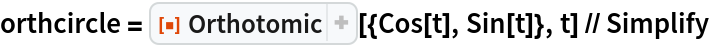Out=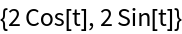In:=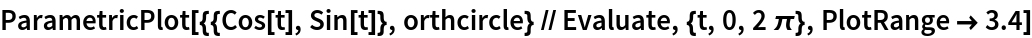Out=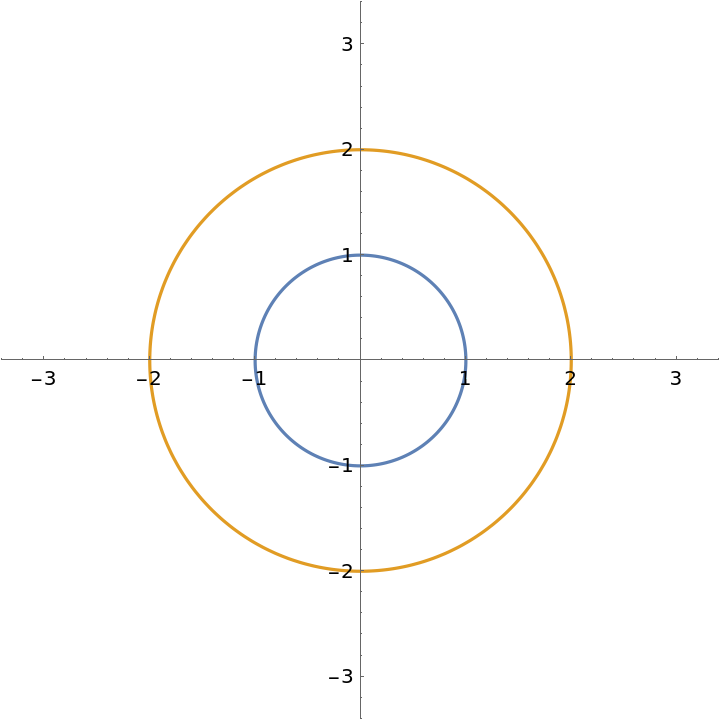### Scope (4)

Orthotomic of a circle with respect to the point {1,1}:

 In:=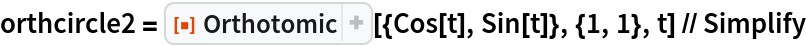Out=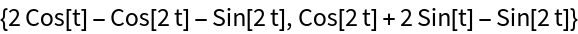In:=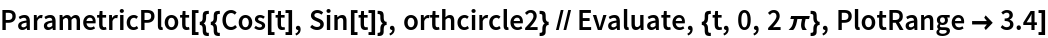Out=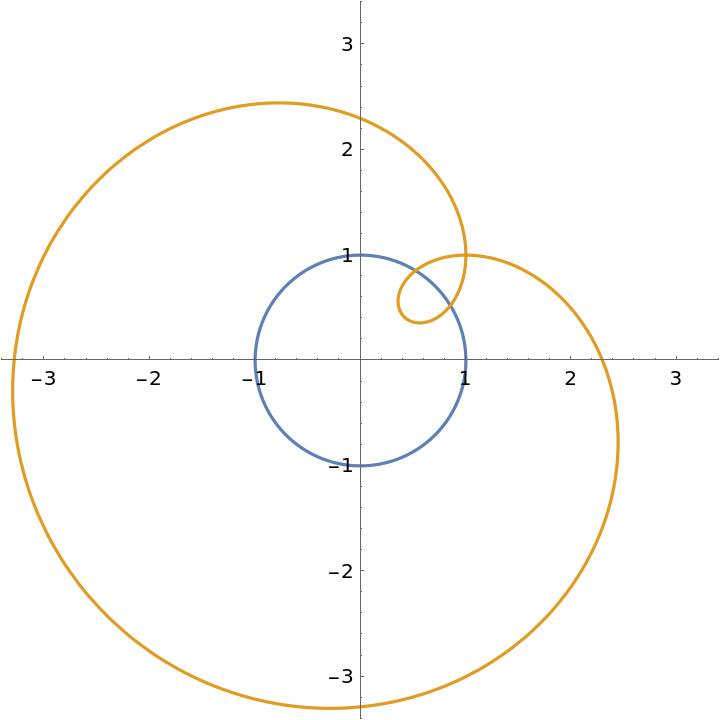Orthotomic of an eight curve with respect to a varying point:

 In:=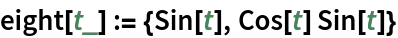In:=Out=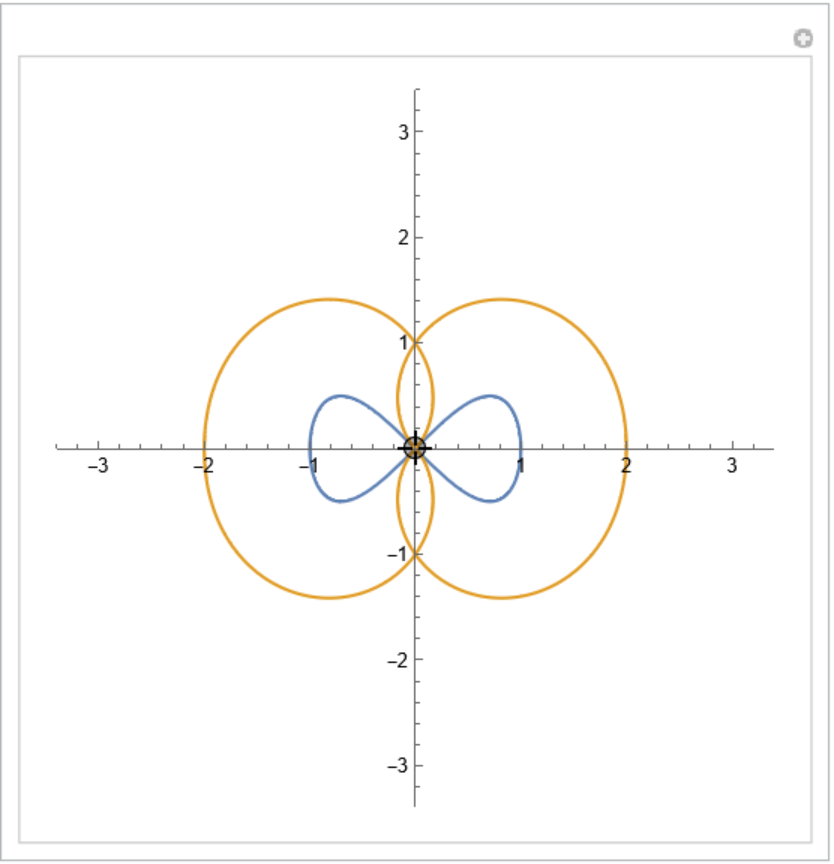Parametric equations for a deltoid:

 In:=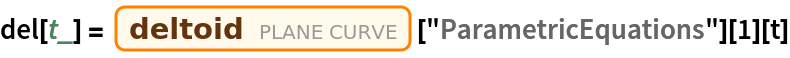Out=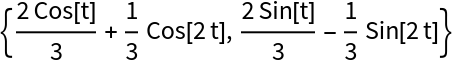Orthotomic of the deltoid with respect to a given line:

 In:=Out=In:=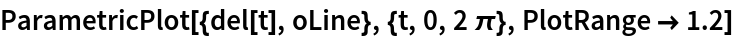Out=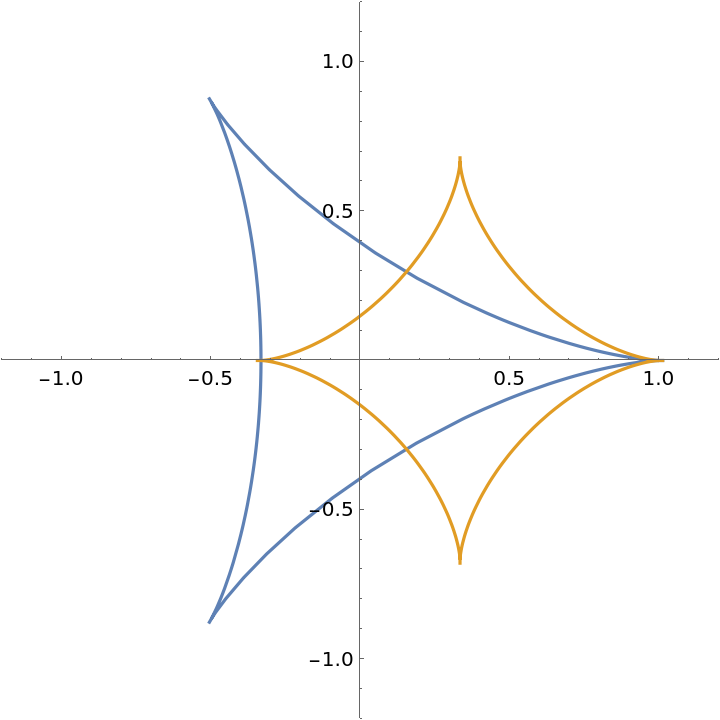Orthotomic of a bifolium with respect to a varying line:

 In:=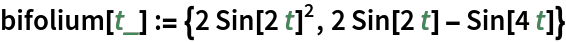In:=Out=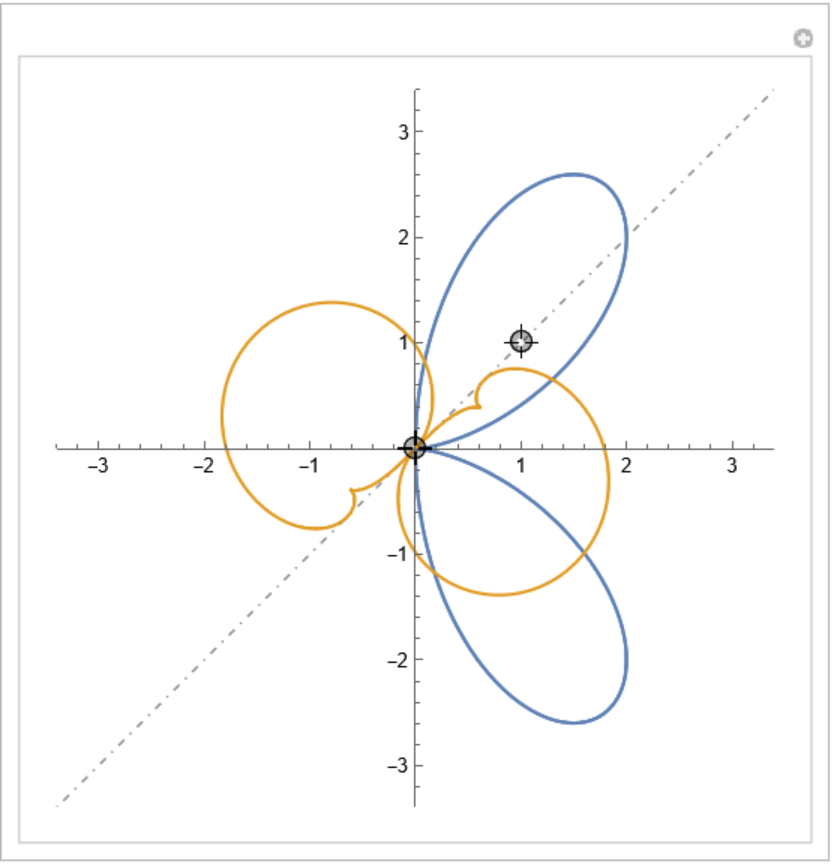### Properties and Relations (2)

The orthotomic is equivalent to the pedal curve, scaled by a factor of 2:

 In:=In:=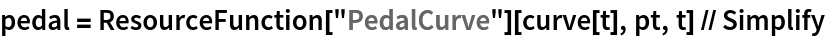Out=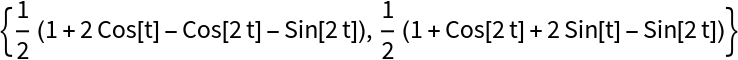In:=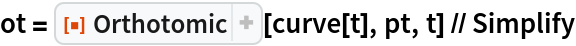Out=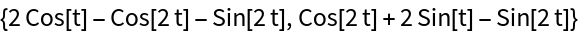In:=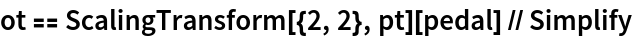Out=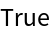In:=Out=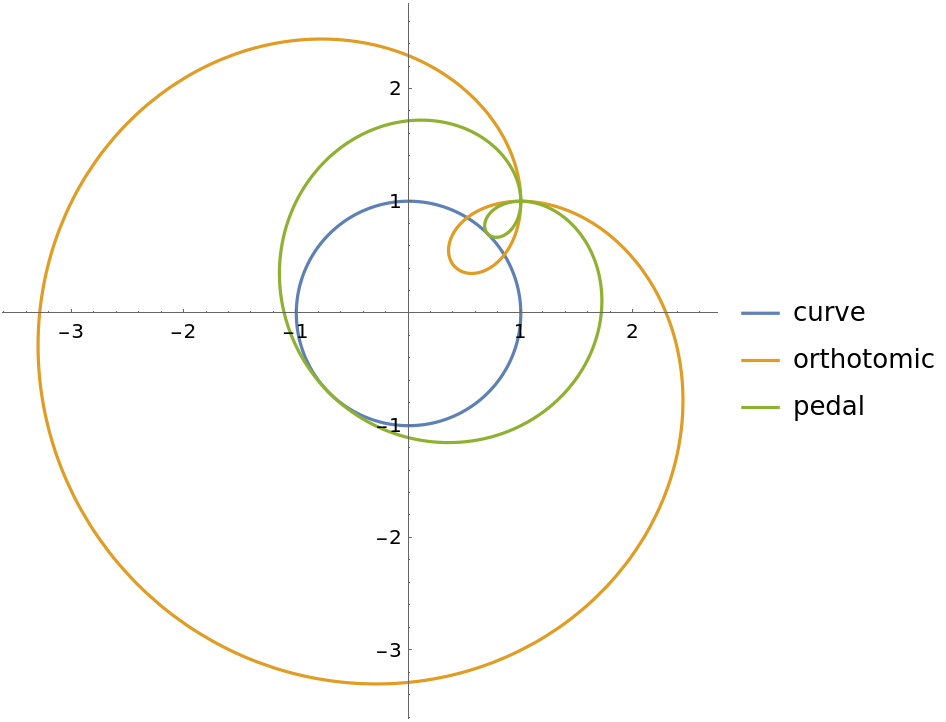The catacaustic curve is the evolute of the orthotomic:

 In:=In:=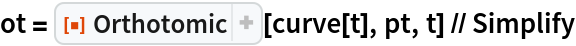Out=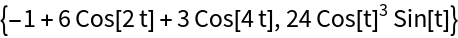In:=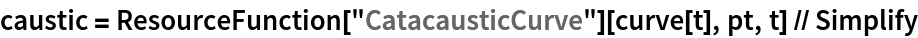Out=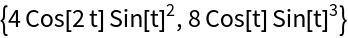In:=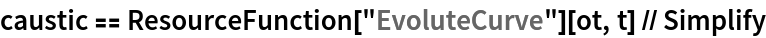Out=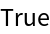In:=Out=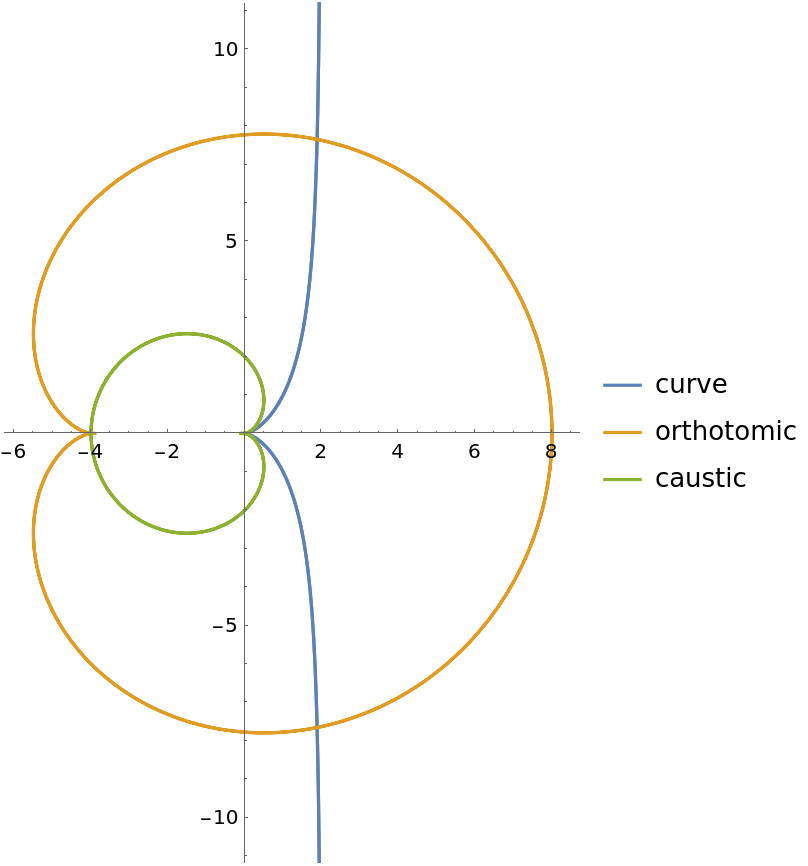### Neat Examples (1)

Generate the orthotomic as an envelope of circles:

 In:=In:=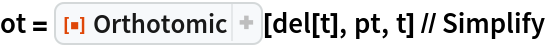Out=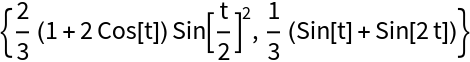In:=Out=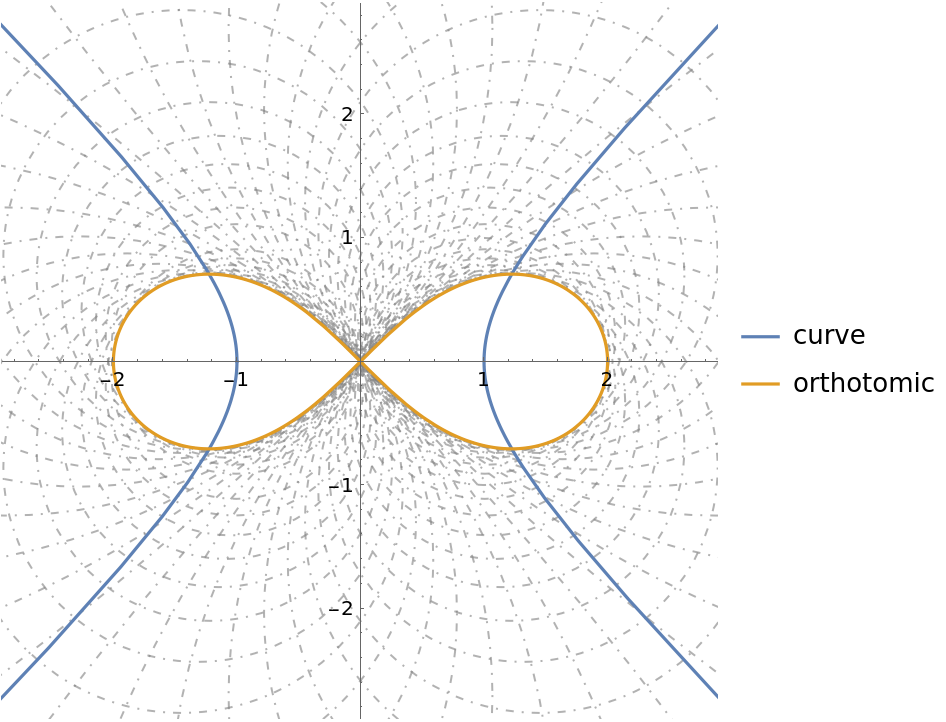Enrique Zeleny

## Version History

• 1.0.1 – 29 March 2022
• 1.0.0 – 15 April 2020